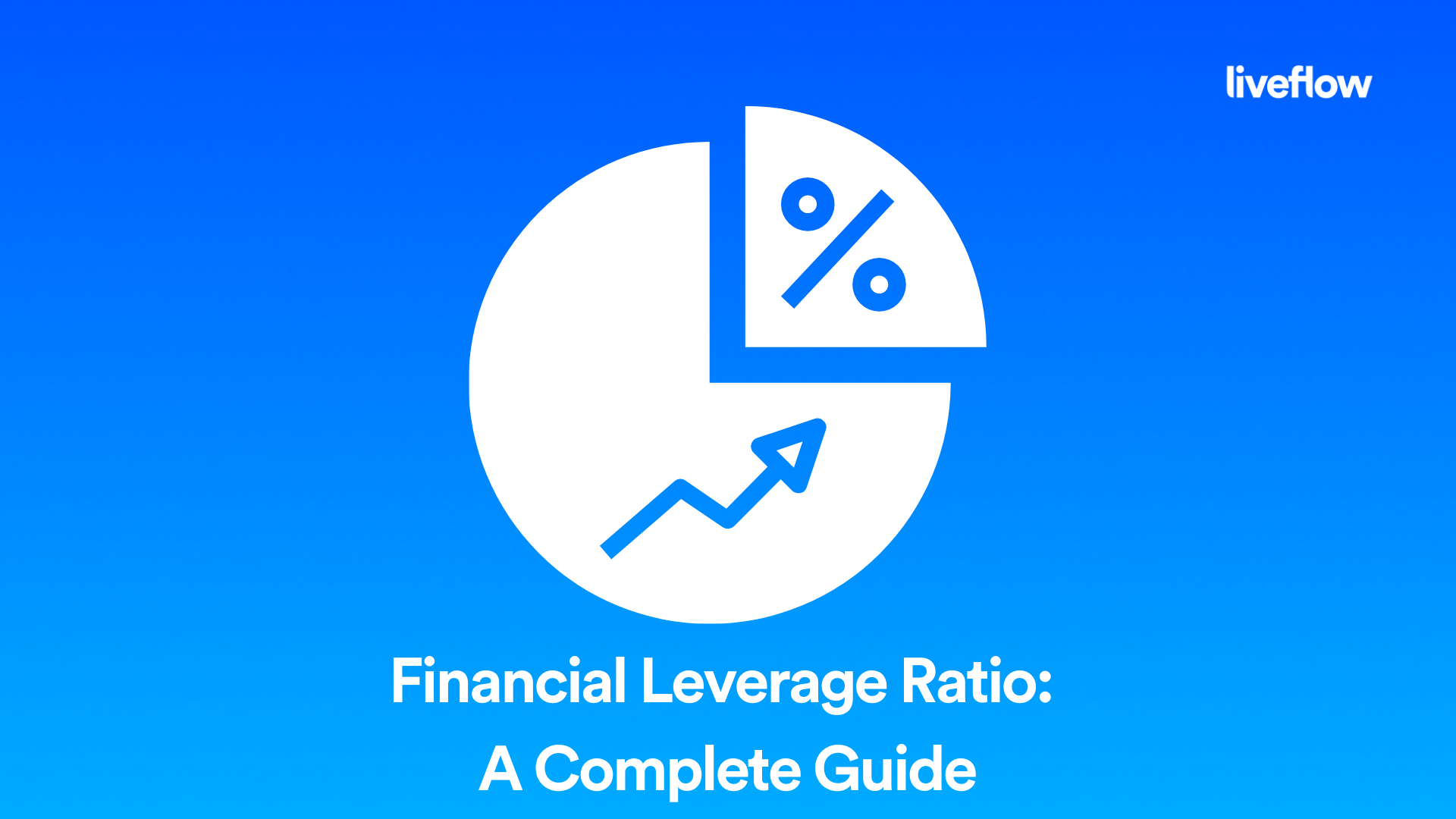# Financial Leverage Ratio: A Complete Guide

July 19, 2022A financial leverage ratio compares a company’s total debt to its total assets. The ratio can be calculated by dividing total liabilities by total assets, or the sum of all long-term debt, short-term debt and capital leases by the sum of all long-term assets and short-term assets.

The financial leverage ratio is used to compare companies with different asset compositions. Companies that have a high proportion of debt can be viewed as more risky than firms with lower levels of debt, since they rely more on borrowed money instead of their own cash flow.

## How is financial leverage ratio calculated?

The financial leverage ratio is also known as the debt-to-equity ratio. It is used to measure the extent to which a company uses borrowed money to fund its operations. It is calculated by dividing total liabilities by total shareholder equity. The higher the ratio, the more debt a company has.

The financial leverage ratio can be used in conjunction with other measures of financial risk, such as liquidity and profitability, to determine whether or not a company's debt levels are too high. If you're considering investing in a company that has a high financial leverage ratio, it's important that you understand exactly why they have so much debt and what they plan to do with it.

## What is the financial leverage ratio formula?

The financial leverage ratio measures the relationship between debt and equity in a company's capital structure. This ratio is also known as the debt-to-equity ratio.

The financial leverage ratio formula is as follows:

Leverage ratio = Total liabilities / Total shareholders' equity

## What does a leverage ratio of 1.5 mean?

A financial leverage ratio is a measure of the amount of debt that a company uses to finance its assets. The higher the ratio, the more debt a company has on its balance sheet. The lower the ratio, the less leverage that a company has.

A financial leverage ratio of 1.5 indicates that a company is using a fair amount of debt to finance its assets. A low leverage ratio would indicate that the company is financing its assets with only equity capital and no debt.

## Summary

Financial leverage ratio is a measure of how much debt a company uses to finance its assets. It's calculated as the total debt divided by the total net worth of the company.

The higher the leverage ratio, the more debt a company has relative to its equity and net worth. The ideal leverage ratio varies from industry to industry and firm to firm, but it's generally accepted that a leverage ratio over 1x is too much debt for most companies.

## Recommendation

LiveFlow is a tool that helps company’s take charge of their financial data and manage bookkeeping with ease. So, if you want to automate you processes and simplify your financial accounting then try LiveFlow today.